# Calculus plots with Makie

The Makie.jl webpage says

From the Jpanese word Maki-e, which is a technique to sprinkle lacquer with gold and silver powder. Data is basically the gold and silver of our age, so let's spread it out beautifully on the screen!

Makie itself is a metapackage for a rich ecosystem. We show how to use the interface provided by AbstractPlotting and the GLMakie backend to produce the familiar graphics of calculus. We do not discuss the MakieLayout package which provides a means to layout multiple graphics and add widgets, such as sliders and buttons, to a layout. We do not discuss MakieRecipes. For Plots, there are "recipes" that make some of the plots more straightforward. We do not discuss the AlgebraOfGraphics which presents an interface for the familiar graphics of statistics. The MakieGallery shows many exmaples of the use of Makie.

## Scenes

Makie draws graphics onto a canvas termed a "scene" in the Makie documentation. There are GLMakie, WGLMakie, and CairoMakie backends for different types of canvases. In the following, we have used GLMakie. WGLMakie is useful for incorporating Makie plots into web-based technologies.

We begin by loading our two packages:

using AbstractPlotting
using GLMakie
#using WGLMakie; WGLMakie.activate!()
#AbstractPlotting.set_theme!(scale_figure=false, resolution = (480, 400))


The Makie developers have workarounds for the delayed time to first plot, but without utilizing these the time to load the package is lengthy.

A scene is produced with Scene() or through a plotting primitive:

scene = Scene()We see next how to move beyond the blank canvas.

## Points (scatter)

The task of plotting the points, say $(1,2)$, $(2,3)$, $(3,2)$ can be done different ways. Most plotting packages, and Makie is no exception, allow the following: form vectors of the $x$ and $y$ values then plot those with scatter:

xs = [1,2,3]
ys = [2,3,2]
scatter(xs, ys)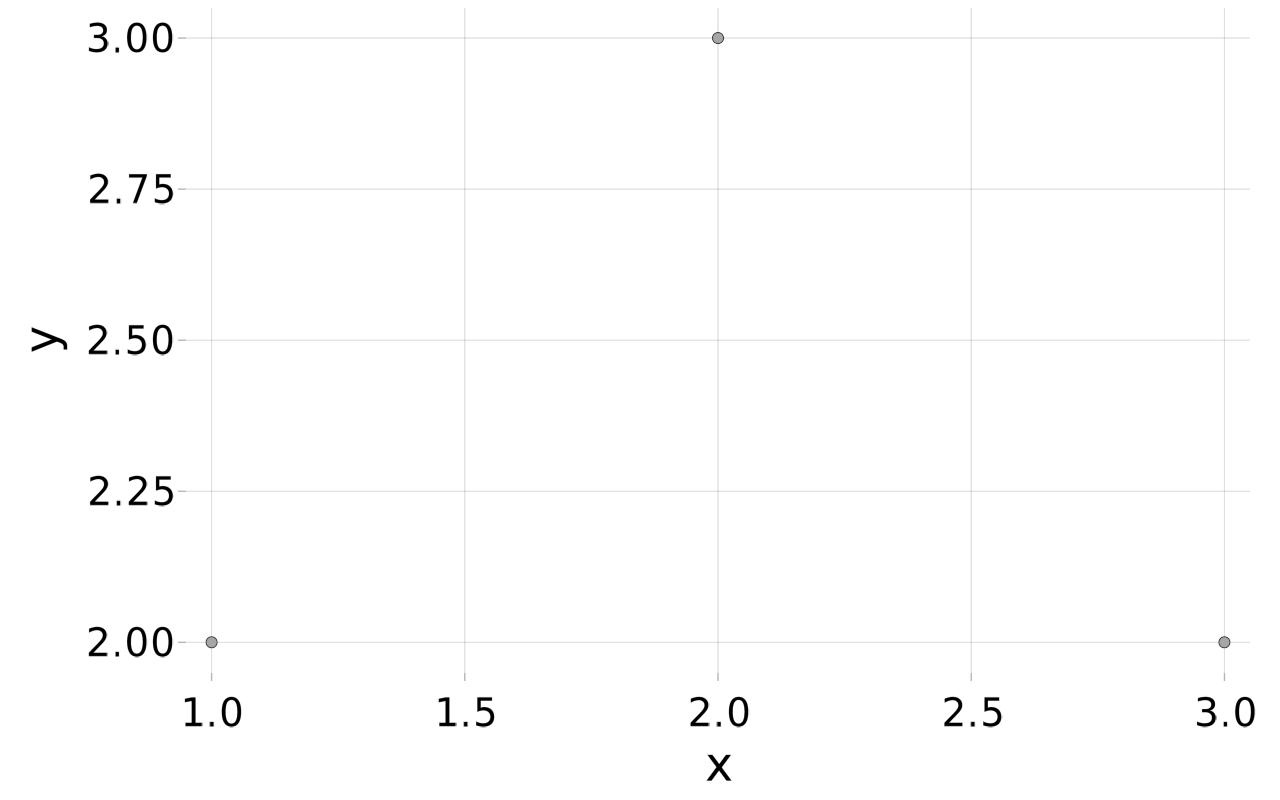The scatter function creates and returns a Scene object, which when displayed shows the plot.

The more generic plot function can also be used for this task.

### Point2, Point3

When learning about points on the Cartesian plane, a "t"-chart is often produced:

x | y
-----
1 | 2
2 | 3
3 | 2

The scatter usage above used the columns. The rows are associated with the points, and these too can be used to produce the same graphic. Rather than make vectors of $x$ and $y$ (and optionally $z$) coordinates, it is more idiomatic to create a vector of "points." Makie utilizes a Point type to store a 2 or 3 dimensional point. The Point2 and Point3 constructors will be utilized.

Makie uses a GPU, when present, to accelerate the graphic rendering. GPUs employ 32-bit numbers. Julia uses an f0 to indicate 32-bit floating points. Hence the alternate types Point2f0 to store 2D points as 32-bit numbers and Points3f0 to store 3D points as 32-bit numbers are seen in the documentation for Makie.

We can plot vector of points in as direct manner as vectors of their coordinates:

pts = [Point2(1,2), Point2(2,3), Point2(3,2)]
scatter(pts)A typical usage is to generate points from some vector-valued function. Say we have a parameterized function r taking $R$ into $R^2$ defined by:

r(t) = [sin(t), cos(t)]

r (generic function with 1 method)


Then broadcasting values gives a vector of vectors, each identified with a point:

ts = [1,2,3]
r.(ts)

3-element Array{Array{Float64,1},1}:
[0.8414709848078965, 0.5403023058681398]
[0.9092974268256817, -0.4161468365471424]
[0.1411200080598672, -0.9899924966004454]


We can broadcast Point2 over this to create a vector of Point objects:

pts = Point2.(r.(ts))

3-element Array{GeometryBasics.Point{2,Float64},1}:
[0.8414709848078965, 0.5403023058681398]
[0.9092974268256817, -0.4161468365471424]
[0.1411200080598672, -0.9899924966004454]


These then can be plotted directly:

scatter(pts)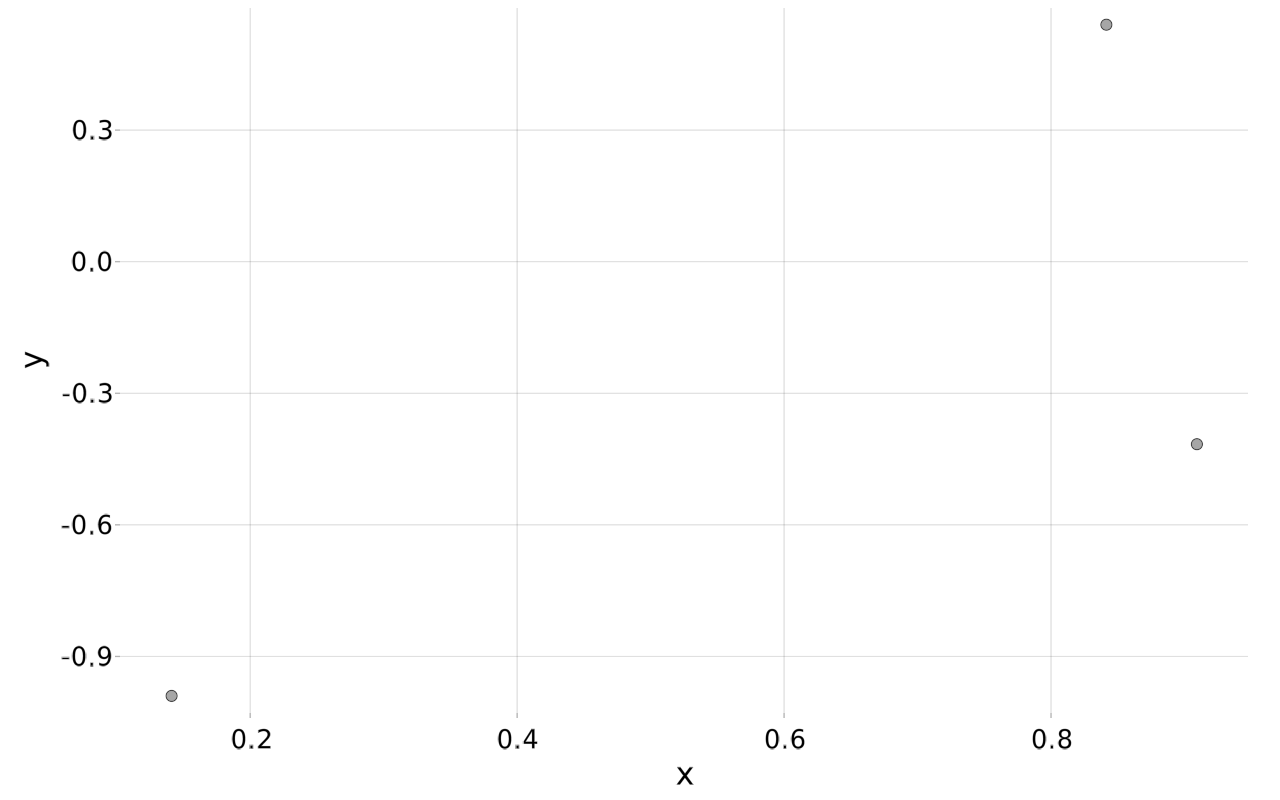The ploting of points in three dimesions is essentially the same, save the use of Point3 instead of Point2.

r(t) = [sin(t), cos(t), t]
ts = range(0, 4pi, length=100)
pts = Point3.(r.(ts))
scatter(pts)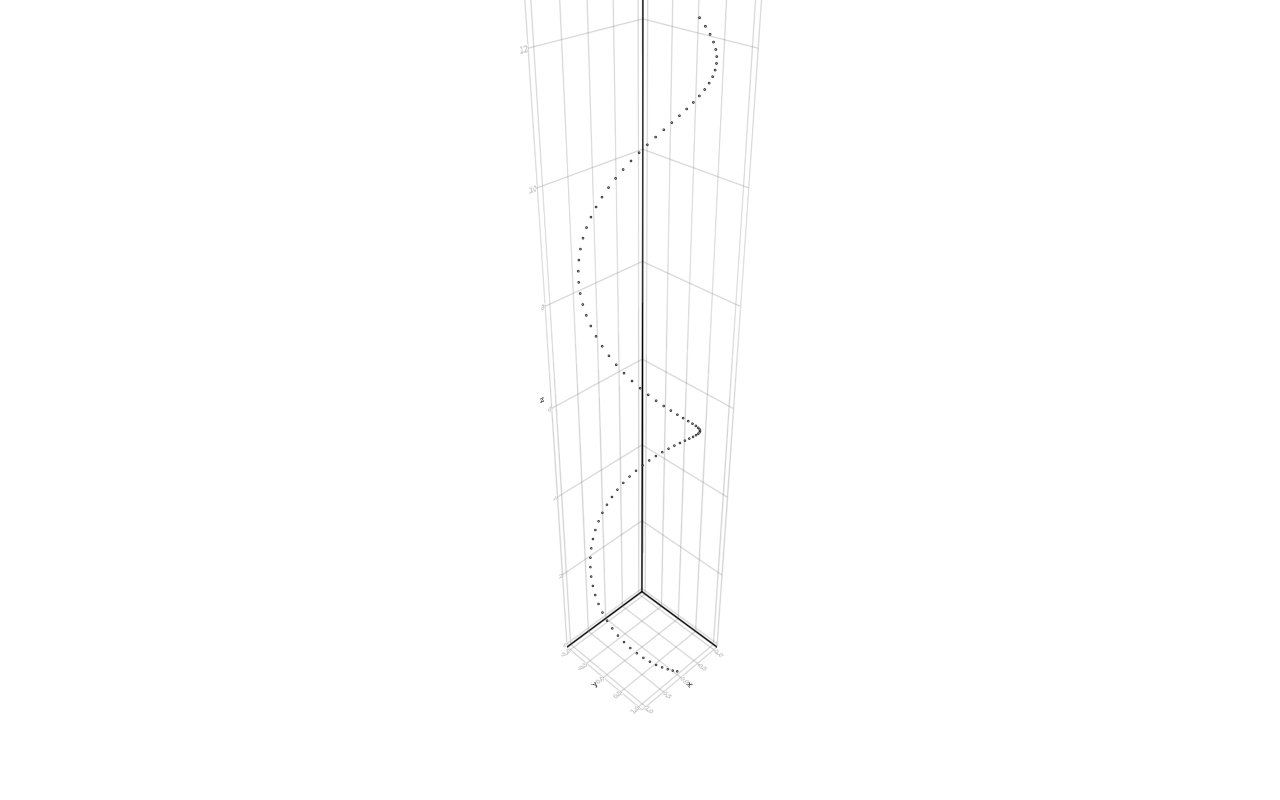To plot points generated in terms of vectors of coordinates, the component vectors must be created. The "t"-table shows how, simply loop over each column and add the corresponding $x$ or $y$ (or $z$) value. This utility function does exactly that, returning the vectors in a tuple.

unzip(vs) = Tuple([vs[j][i] for j in eachindex(vs)] for i in eachindex(vs))

unzip (generic function with 1 method)


(The functionality is essentially a reverse of the zip function, hence the name.)

We might have then:

scatter(unzip(r.(ts))...)where splatting is used to specify the xs, ys, and zs to scatter.

(Compare to scatter(Point3.(r.(ts))) or scatter(Point3∘r).(ts)).)

### Attributes

A point is drawn with a "marker" with a certain size and color. These attributes can be adjusted, as in the following:

scatter(xs, ys, marker=[:x,:cross, :circle], markersize=25, color=:blue)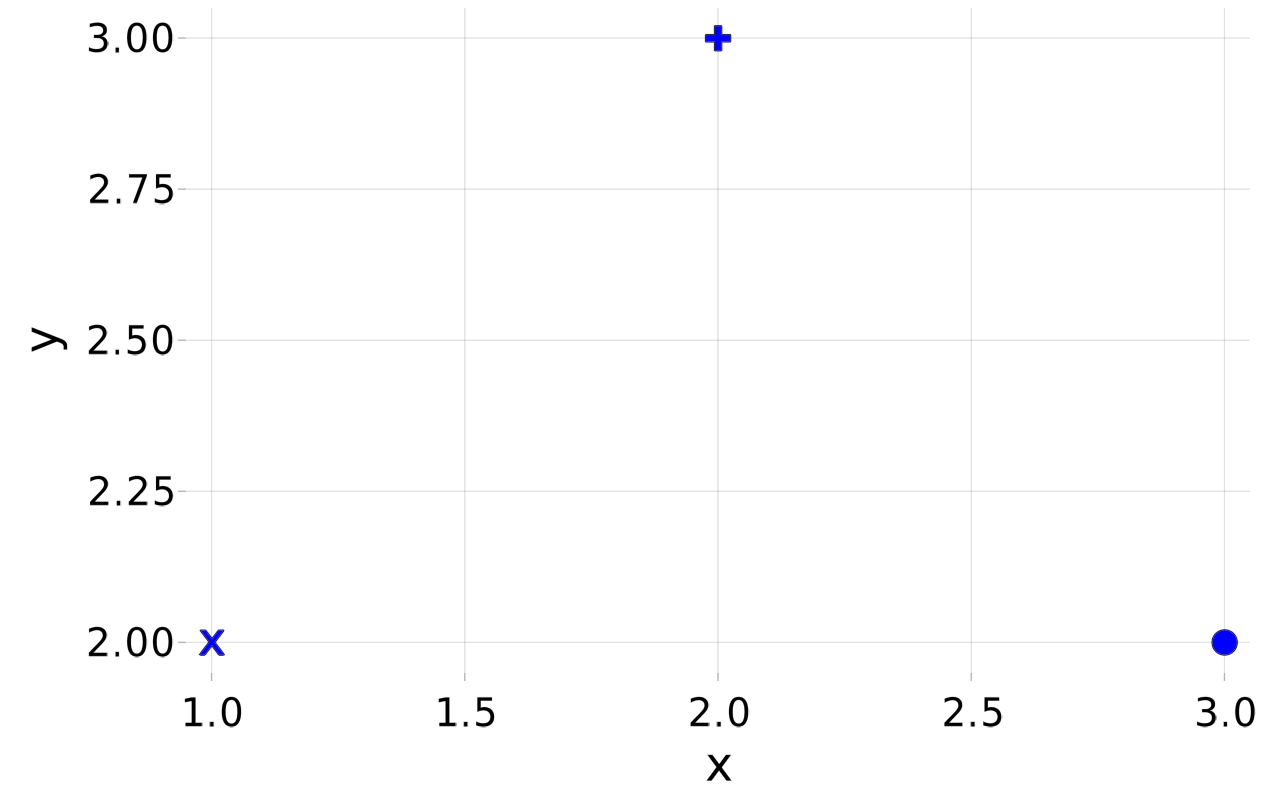Marker attributes include

• marker a symbol, shape. A single value will be repeated. A vector of values of a matching size will specify a marker for each point.

• marker_offset offset coordinates

• markersize size (radius pixels) of marker

### Text (text)

Text can be placed at a point, as a marker is. To place text the desired text and a position need to be specified.

For example:

pts = Point2.(1:5, 1:5)
scene = scatter(pts)
[text!(scene, "text", position=pt, textsize=1/i, rotation=2pi/i) for (i,pt) in enumerate(pts)]
scene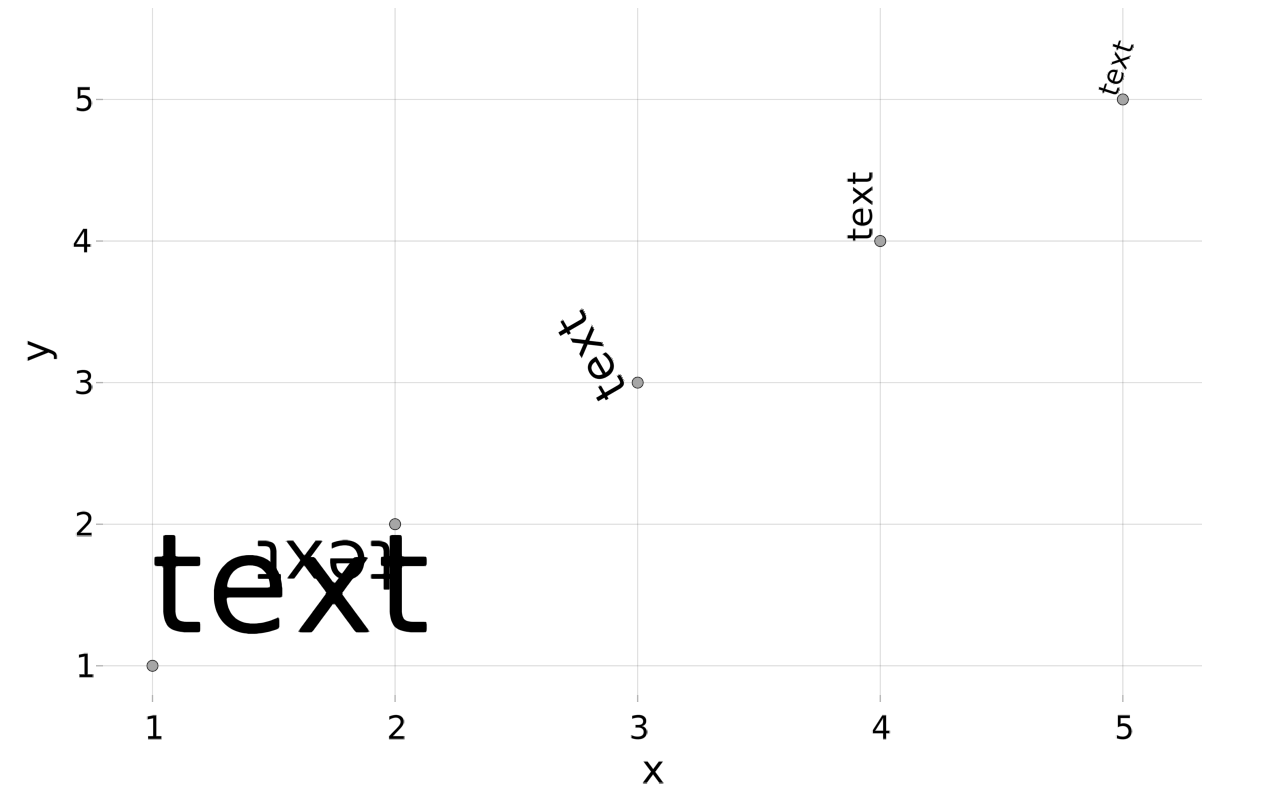The graphic shows that position positions the text, textsize adjusts the displayed size, and rotation adjusts the orientation.

Attributes for text include:

• position to indicate the position. Either a Point object, as above, or a tuple

• align Specify the text alignment through (:pos, :pos), where :pos can be :left, :center, or :right.

• rotation to indicate how the text is to be rotated

• textsize the font point size for the text

• font to indicate the desired font

## Curves

### Plots of univariate functions

The basic plot of univariate calculus is the graph of a function $f$ over an interval $[a,b]$. This is implemented using a familiar strategy: produce a series of representative values between $a$ and $b$; produce the corresponding $f(x)$ values; plot these as points and connect the points with straight lines. The lines function of AbstractPlotting will do the last step.

By taking a sufficient number of points within $[a,b]$ the connect-the-dot figure will appear curved, when the function is.

To create regular values between a and b either the range function, the related LinRange function, or the range operator (a:h:b) are employed.

For example:

f(x) = sin(x)
a, b = 0, 2pi
xs = range(a, b, length=250)
lines(xs, f.(xs))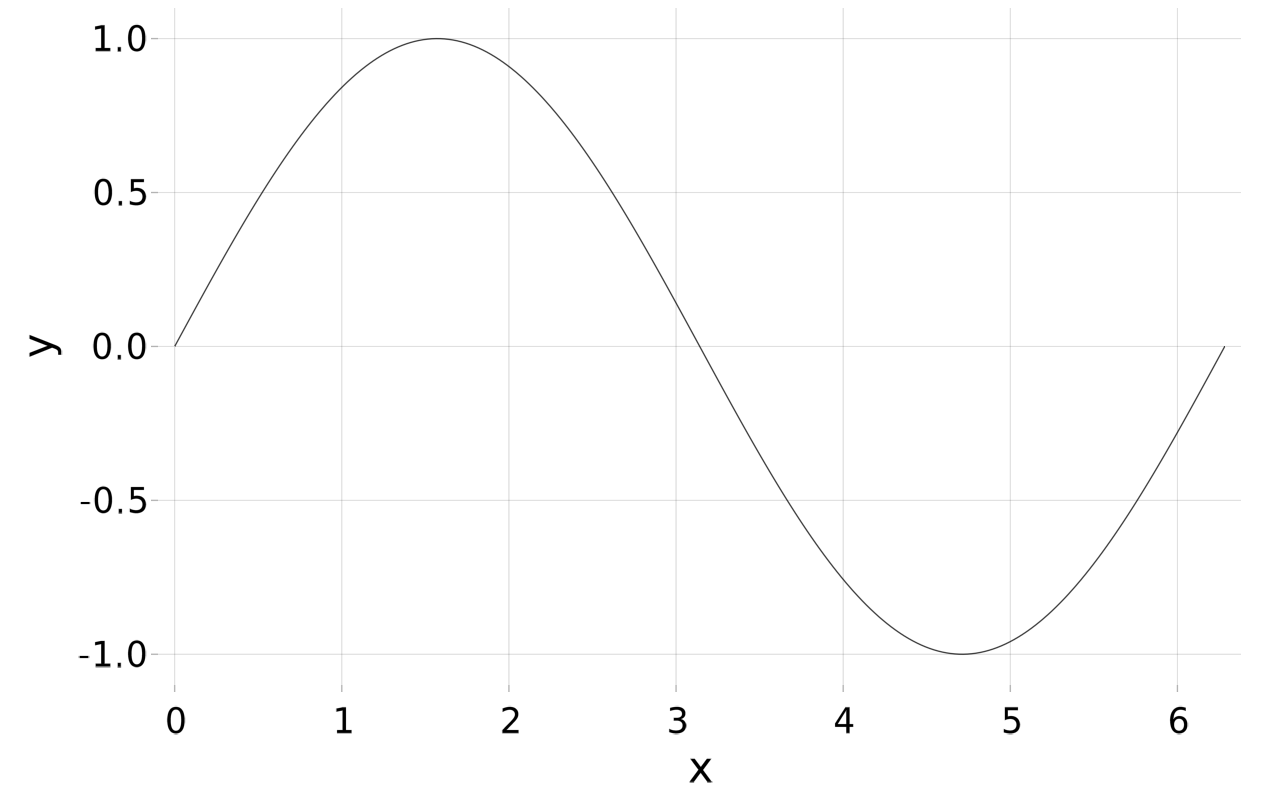Or

f(x) = cos(x)
a, b = -pi, pi
xs = a:pi/100:b
lines(xs, f.(xs))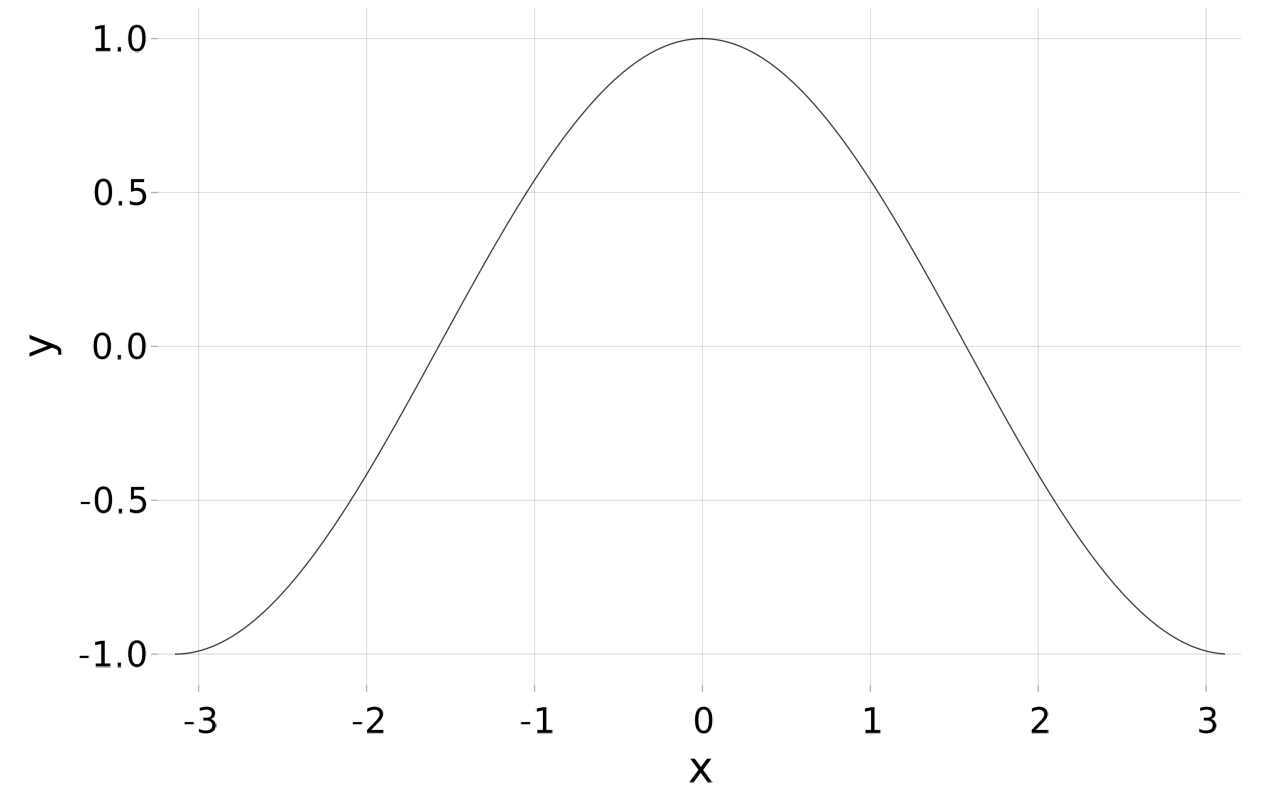As with scatter, lines returns a Scene object that produces a graphic when displayed.

As with scatter, lines can can also be drawn using a vector of points:

lines([Point2(x, fx) for (x,fx) in zip(xs, f.(xs))])

(Though the advantage isn't clear here, this will be useful when the points are more naturally generated.)

When a y value is NaN or infinite, the connecting lines are not drawn:

xs = 1:5
ys = [1,2,NaN, 4, 5]
lines(xs, ys)

As with other plotting packages, this is useful to represent discontinuous functions, such as what occurs at a vertical asymptote.

#### Adding to a scene (lines!, scatter!, ...)

To add or modify a scene can be done using a mutating version of a plotting primitive, such as lines! or scatter!. The names follow Julia's convention of using an ! to indicate that a function modifies an argument, in this case the scene.

Here is one way to show two plots at once:

xs = range(0, 2pi, length=100)
scene = lines(xs, sin.(xs))
lines!(scene, xs, cos.(xs))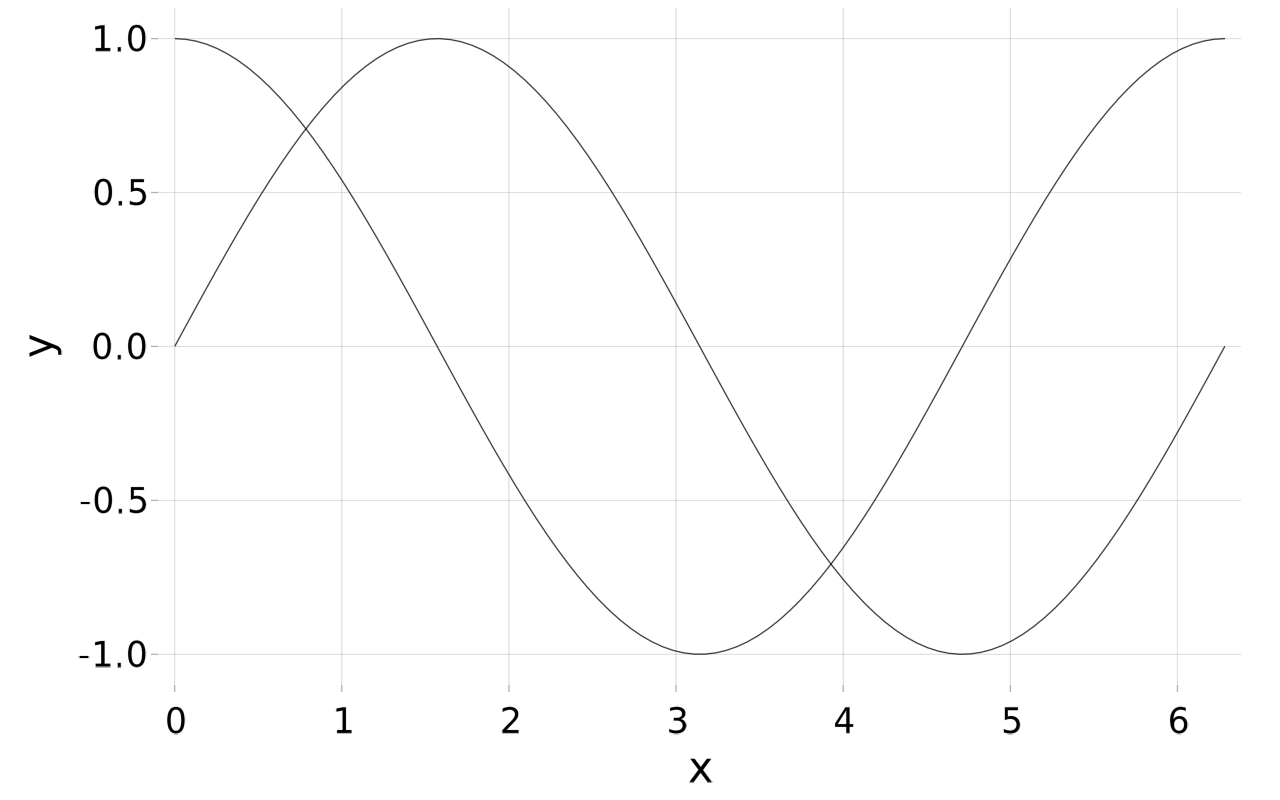We will see soon how to modify the line attributes so that the curves can be distinguished.

The following shows the construction details in the graphic, and that the initial scene argument is implicitly assumed:

xs = range(0, 2pi, length=10)
lines(xs, sin.(xs))
scatter!(xs, sin.(xs), markersize=10)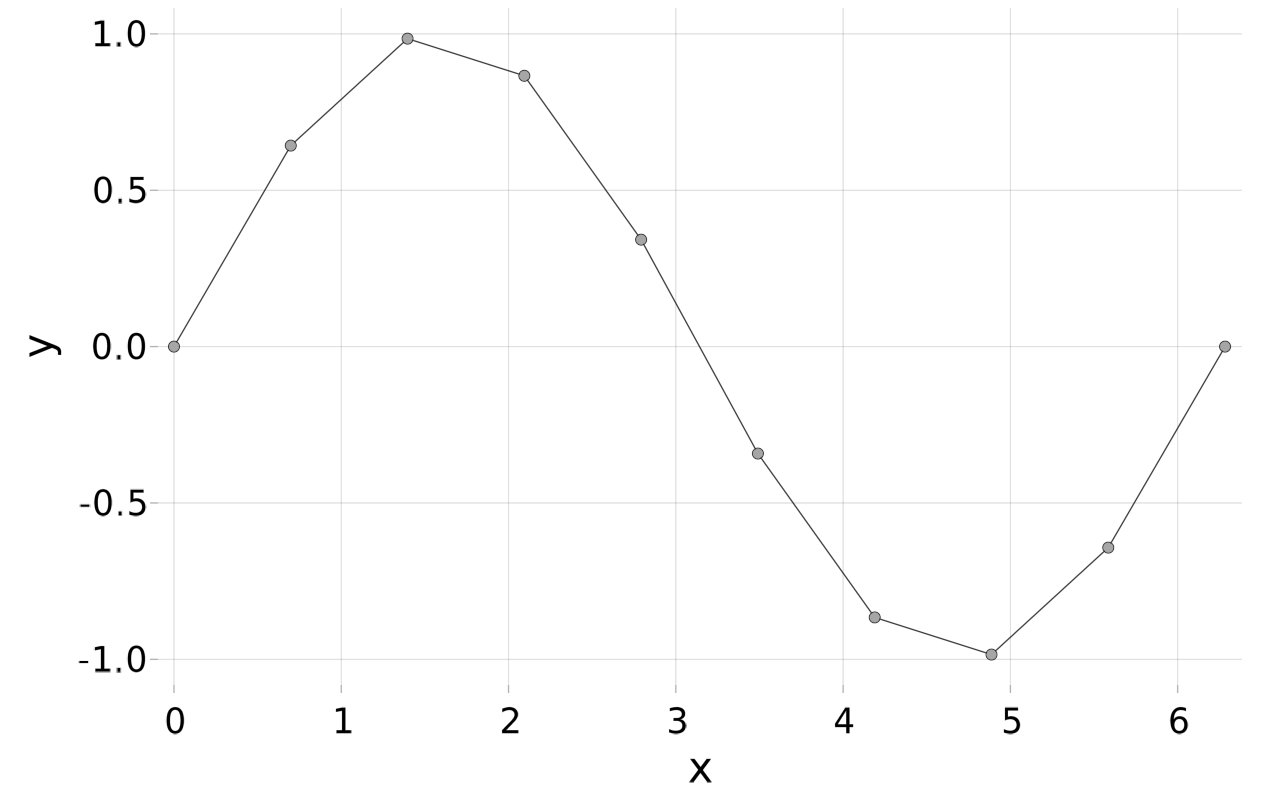The current scene will have data limits that can be of interest. The following indicates how they can be manipulated to get the limits of the displayed x values.

xs = range(0, 2pi, length=200)
scene = plot(xs, sin.(xs))
rect = scene.data_limits[] # get limits for g from f
a, b = rect.origin,  rect.origin + rect.widths

(-0.9633175f0, 6.2831855f0)


In the output it can be discerned that the values are 32-bit floating point numbers and yield a slightly larger interval than specified in xs.

As an example, this shows how to add the tangent line to a graph. The slope of the tangent line being computed by ForwardDiff.derivative.

using ForwardDiff
f(x) = x^x
a, b= 0, 2
c = 0.5
xs = range(a, b, length=200)

tl(x) = f(c) + ForwardDiff.derivative(f, c) * (x-c)

scene = lines(xs, f.(xs))
lines!(scene, xs, tl.(xs), color=:blue)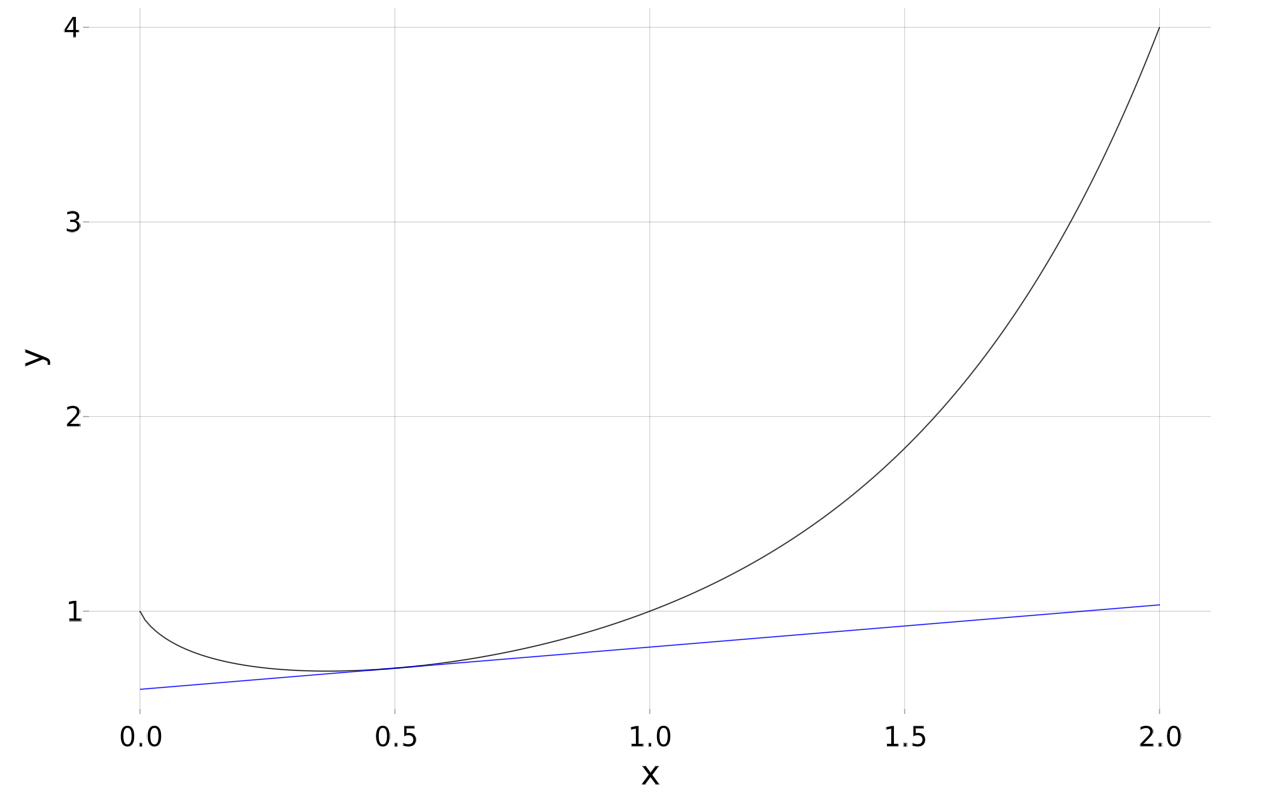#### Attributes

In the last example, we added the argument color=:blue to the lines! call. This set an attribute for the line being drawn. Lines have other attributes that allow different ones to be distinguished, as above where colors indicate the different graphs.

Other attributes can be seen from the help page for lines, and include:

• color set with a symbol, as above, or a string

• linestyle available styles are set by a symbol, one of :dash, :dot, :dashdot, or :dashdotdot.

• linewidth width of line

• transparency the alpha value, a number between $0$ and $1$, smaller numbers for more transparent.

A legend can also be used to help identify different curves on the same graphic, though this is not illustrated. There are examples in the Makie gallery.

#### Scene attributes

Attributes of the scene include any titles and labels, the limits that define the coordinates being displayed, the presentation of tick marks, etc.

The title function can be used to add a title to a scene. The calling syntax is title(scene, text).

To set the labels of the graph, there are "shorthand" functions xlabel!, ylabel!, and zlabel!. The calling pattern would follow xlabel!(scene, "x-axis").

The plotting ticks and their labels are returned by the unexported functions tickranges and ticklabels. The unexported xtickrange, ytickrange, and ztickrange; and xticklabels, yticklabels, and zticklabels return these for the indicated axes.

These can be dynamically adjusted using xticks!, yticks!, or zticks!.

pts = [Point2(1,2), Point2(2,3), Point2(3,2)]
scene = scatter(pts)
title(scene, "3 points")
ylabel!(scene, "y values")
xticks!(scene, xtickrange=[1,2,3], xticklabels=["a", "b", "c"])


To set the limits of the graph there are shorthand functions xlims!, ylims!, and zlims!. This might prove useful if vertical asymptotes are encountered, as in this example:

f(x) = 1/x
a,b = -1, 1
xs = range(-1, 1, length=200)
scene = lines(xs, f.(xs))
ylims!(scene, (-10, 10))
center!(scene)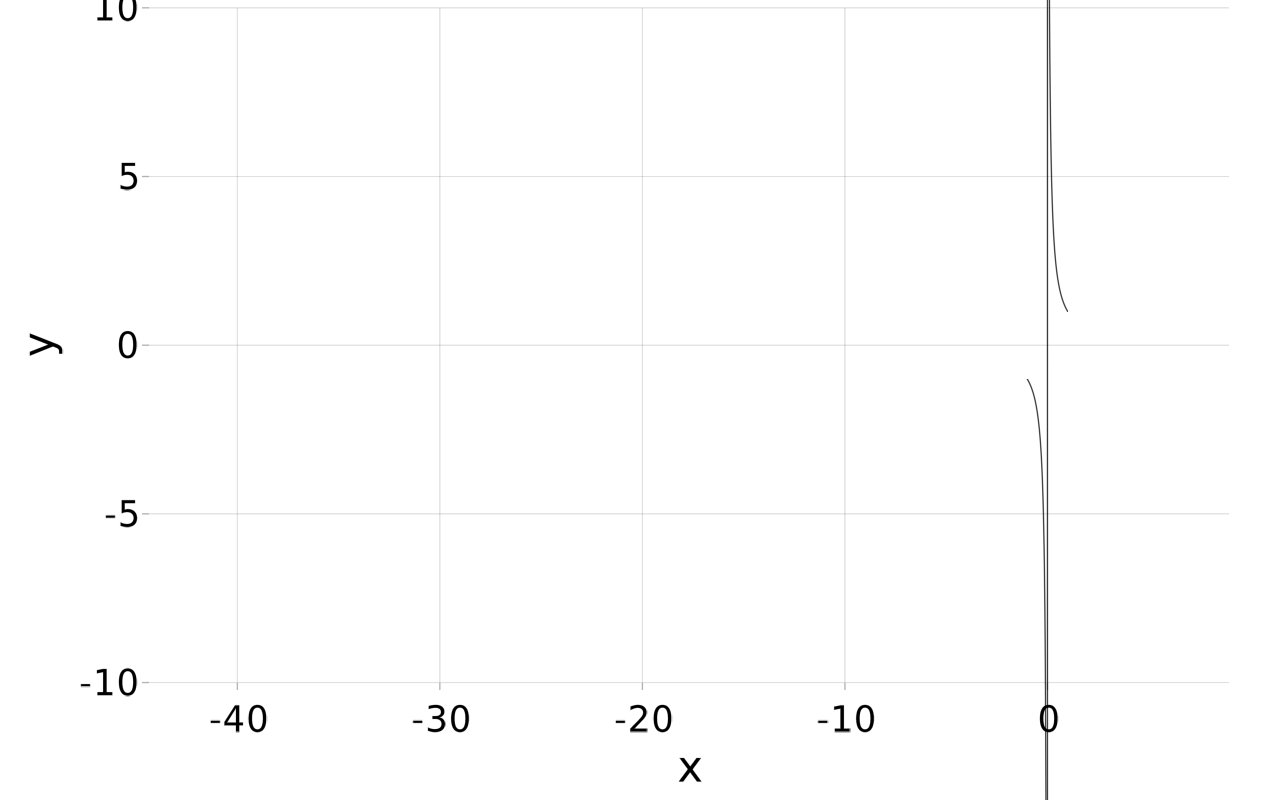### Plots of parametric functions

A space curve is a plot of a function $f:R^2 \rightarrow R$ or $f:R^3 \rightarrow R$.

To construct a curve from a set of points, we have a similar pattern in both $2$ and $3$ dimensions:

r(t) = [sin(2t), cos(3t)]
ts = range(0, 2pi, length=200)
pts = Point2.(r.(ts))  # or (Point2∘r).(ts)
lines(pts)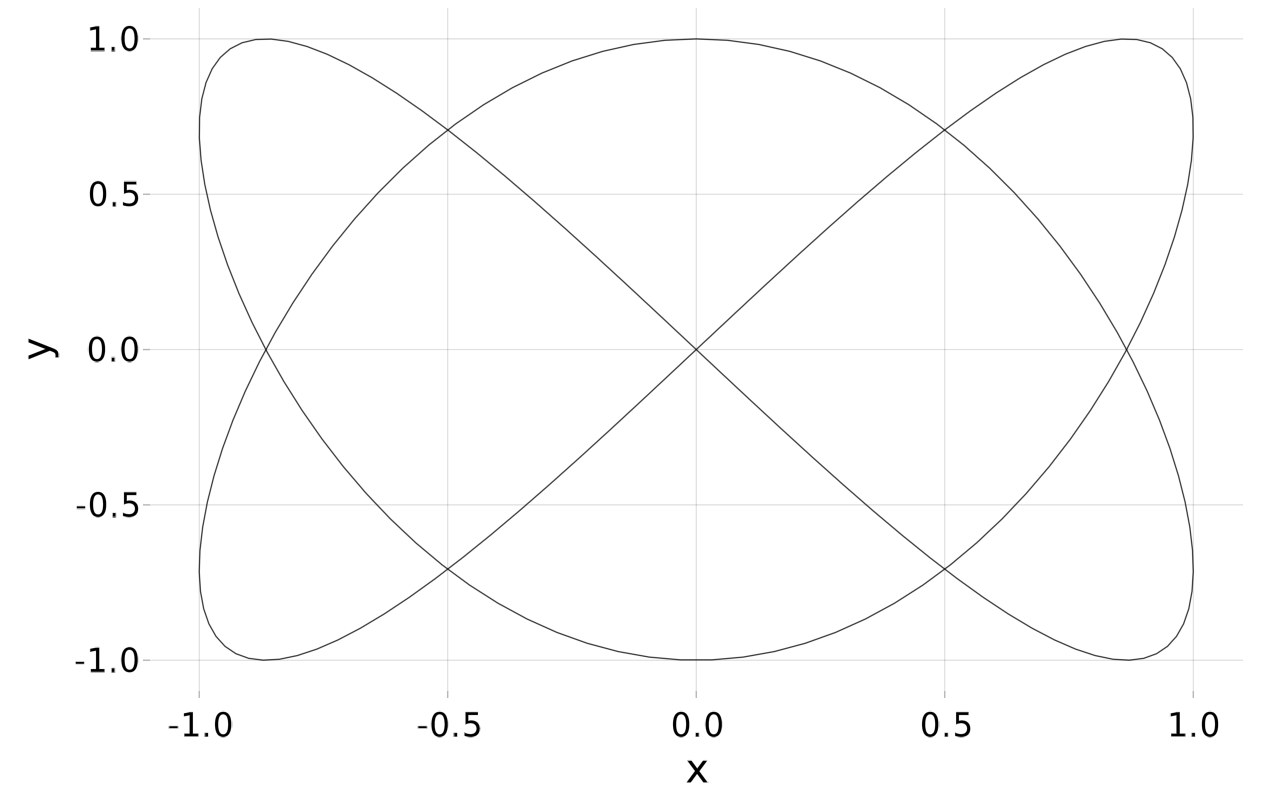Or

r(t) = [sin(2t), cos(3t), t]
ts = range(0, 2pi, length=200)
pts = Point3.(r.(ts))
lines(pts)